April 3, 2014

## What is Dynamic Document?

Recall how you finished a STAT 526 Homework:

• Write a piece of code
• Run the code to get output and graph
• Copy and paste them to your document processor
• Repeat steps above

Can we make life a little bit easier?

• Human language and code in the same document
• Code can be excuted and replaced by its output
• Focus more on content, less on format

## A minimal example

Source code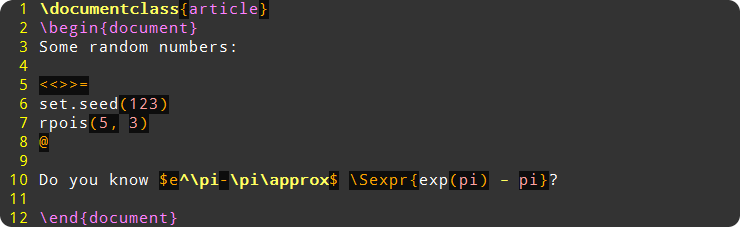After compiling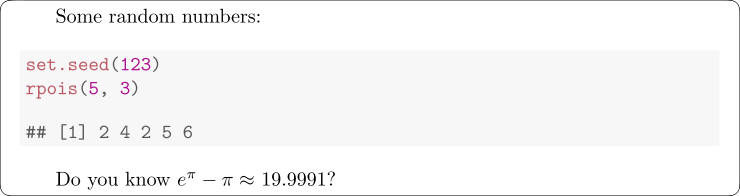## A minimal example (plots)

Source code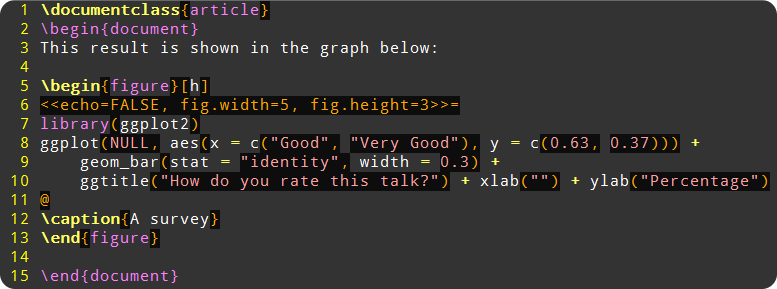## A minimal example (plots)

After compiling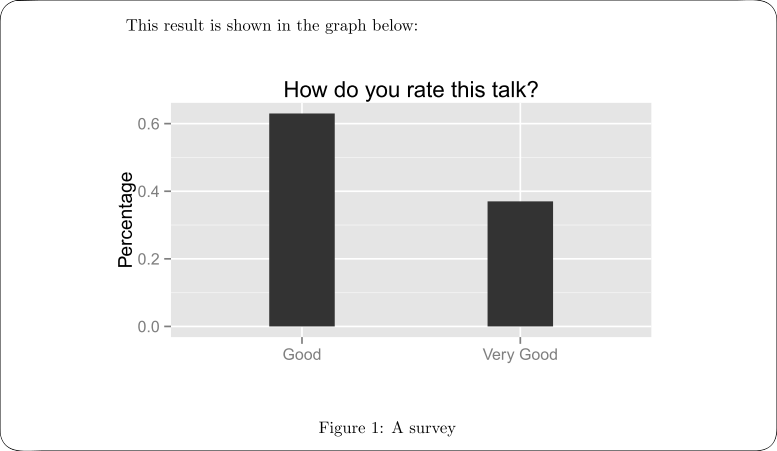## Basic idea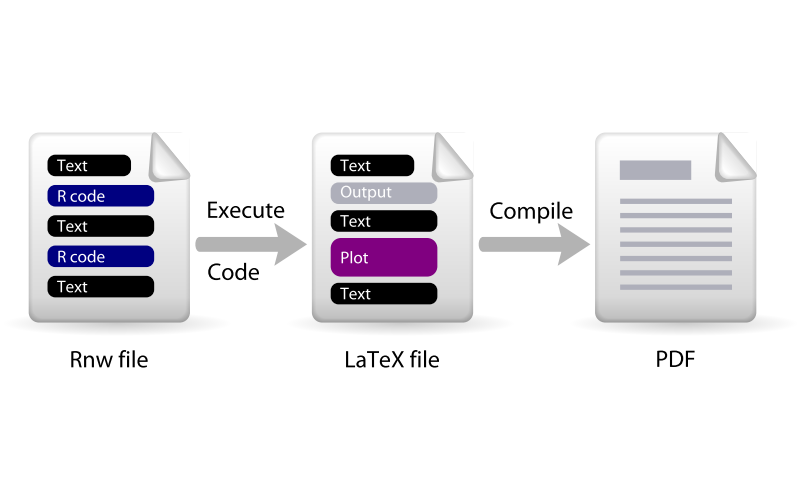## Tools

Early stage – Sweave

• To "weave" S
• Developed by R Core, very powerful

Today – knitr## Structure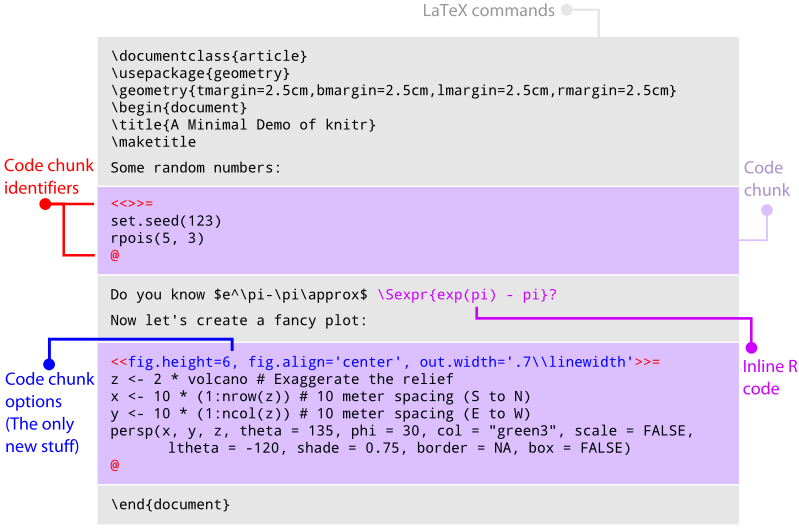## Most useful options (output)

• Default option, show both source code and output
<<>>=
x <- seq(1, 6, by = 0.5)
x
sin(x)
@
• Show R source code only
<<eval=FALSE>>=
@
• Show code output only
<<echo=FALSE>>=
@

## Most useful options (output)

• Whether to format the code or not
<<tidy=FALSE>>=
x<-                 seq(1,6,
by=0.5)
x
@
• Only evaluate the code, do not show anything. Mainly used to create variables for later use.
<<include=FALSE>>=
dat = rnorm(100)
@

## Most useful options (plot)

• Figure width and height (in R side)
<<fig.width=6, fig.height=6>>=
plot(density(dat))
@
• Figure width and height (in output document side)
<<out.width='0.7\\textwidth'>>=
plot(density(dat))
@
• Alignment and caption
<<fig.align='center', fig.cap="A density plot">>=
plot(density(dat))
@

## From $$\LaTeX$$ to Markdown

$$\LaTeX$$ is a powerful language to write document, but…

• Not easy to get started
• Complicated, typing too many commands
• Restricted output file format (mainly for PDF)

Another friend of knitr – Markdown

• So… another language to learn?
• Yes, but…
• Much, much, much easier than $$\LaTeX$$
• Can be converted into $$\LaTeX$$, HTML, Word etc.

## An overview of Markdown+knitr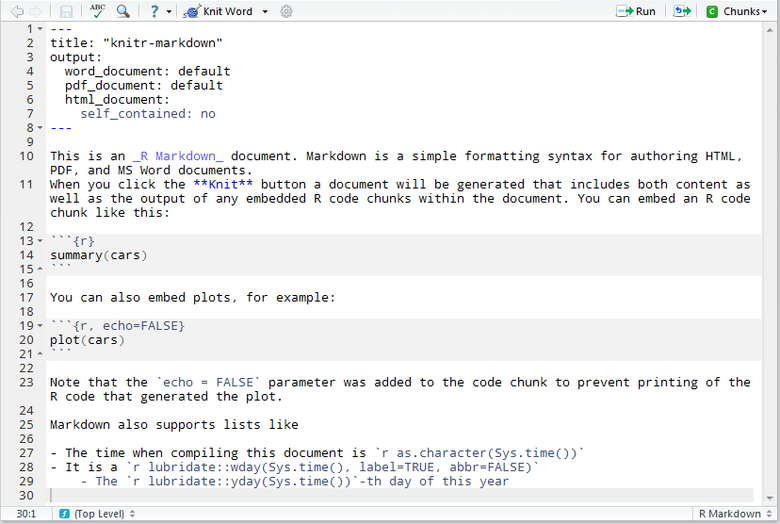## Markdown basics

## Methodology
In this section we introduce our methodology.

### Algorithm
The algorithm is blablabla...

### Simulation
Now we show our simulation result.
• Similar to \section{} and \subsection{} in $$\LaTeX$$

## Markdown basics

• Decorations (bold, italic, etc.)
You should **NEVER** claim to _accept_ a null hypothesis

You just say **_do not reject_**
• Rendered result

You should NEVER claim to accept a null hypothesis

You just say do not reject

## Markdown basics

• Code
There is some inline code: print(x)

And code blocks:

x <- rnorm(100)
summary(x)

• Rendered result

There is some inline code: print(x)

And code blocks:

x <- rnorm(100)
summary(x)

## Markdown basics

• Math formulas
The series $\sum_i \frac{1}{n}$ is divergent, i.e.,
$$\sum_i \frac{1}{n}=\infty$$
• Rendered result

The series $$\sum_i \frac{1}{n}$$ is divergent, i.e.,

$\sum_i \frac{1}{n}=\infty$

## Markdown basics

• Item lists
There are three kinds of lies:

- Lies
- Damned lies
- Statistics
- Frequentists
- Bayesian

There are three kinds of lies:

• Lies
• Damned lies
• Statistics
• Frequentists
• Bayesian

## Markdown basics

[A 5-minute tutorial of Markdown](http://goo.gl/JqhpOf)

The talk is boring, but please wake up! ![](images/tuzki31.gif)
• Rendered result

A 5-minute tutorial of Markdown

The talk is boring, but please wake up!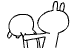## knitr with Markdown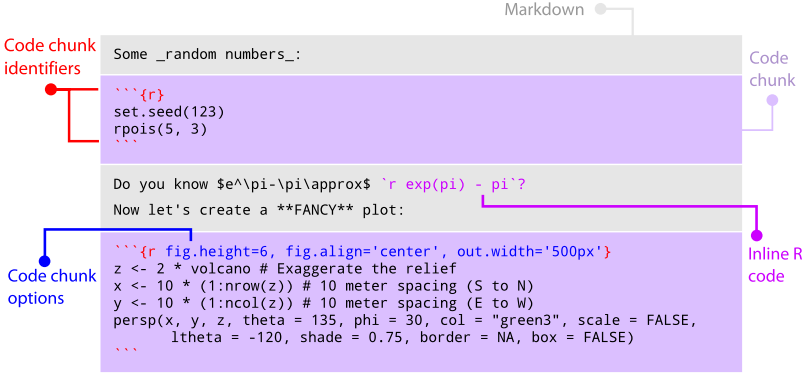## More expressive, more impressive

• 3-D interactive plots
• Animation
• Slides
• Did I tell you this slide was written using Markdown+knitr?
• More data analysis reports at RPubs
• More visualization examples at Vistat

## AcknowledgementThanks to Yihui Xie, the creator of knitr, for creating this wonderful tool, and for providing me lots of help in using knitr.